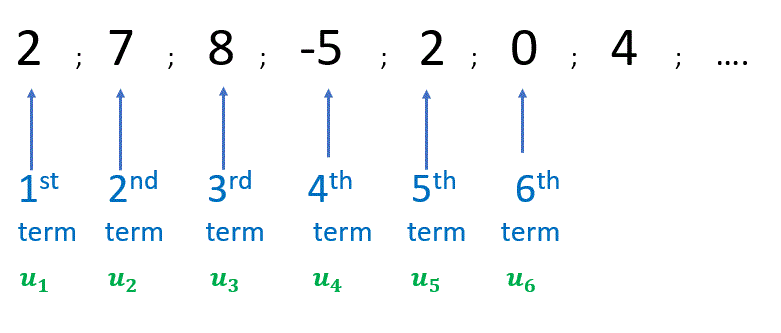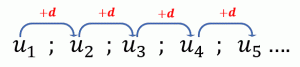Select Page

# Arithmetic sequences

Objectives: :

• – Define arithmetic sequences and series.
• – Use of the formulae for the nth term and the sum of the first n terms of the sequence.
• – Use of sigma notation for sums of arithmetic sequences.

## SEQUENCE

### A sequence is just an ordered list of numbers (terms in a definite order). For example :## SIGMA NOTATION $$\sum\limits_{n=1}^{k}$$

### Examples :## Definition:

### Arithmetic sequences are characterized by the fact that to get from one term to the next we always add the same amount.### The common difference $$d$$

#### If $$u_1 =10, d=-3$$ the sequence is $$10 ; 7 ; 4 ; 1 ; -2 ; …$$### where $$a_1$$ is the first term and $$d$$ the common difference.### $$d$$, the common difference.

#### – test